# Demystifying Probability, Part 2

In the previous article, I introduced Rustywolf’s Probability Calculator and we saw the calculator in action. In this article, we delve into the maths behind it.

Binomial Coefficients
First, we need to understand what a Binomial Coefficient is. Don’t be put off by the fancy mathematical term, it’s a very concrete and easy to understand object.

A binomial coefficient is “the number of ways we can pick things out of a group.” They look like this: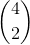You read it as “4 choose 2”. And that’s exactly what it means: it’s the number of ways of choosing 2 things out of a group of 4.

What does this look like? Let’s take 4 cards, A, B, C, D. Let’s write out the number of different combinations of 2 cards there are.

AB
AC
BC
BD
CD

That’s all of the possible combinations. Any other combination would just be one of the above, but maybe in a different order. So “4 choose 2” = 6, because there are 6 ways of choosing 2 cards out of 4.

There is a mathematical formula for calculating the value of a binomial coefficient, but we won’t need it. If we want to calculate any, we can ask Google directly:

http://prnt.sc/b713x3

Google is great. It makes a convenient calculator as we can type any equations we need directly into it.

Similarly we can calculate the number of possible 5-card hands of a 40-card deck. We want the value of “40 choose 5”, or in maths notation,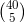:

http://prnt.sc/ba8325

Of course, if we’re running multiple copies of a particular card, some of these hands would look the same.

The Hypergeometric Distribution
‘Hypergeometric Distribution’ is, as far as we’re concerned, just a fancy mathematical term for the maths describing drawing cards from a deck.

It’ll be easiest to see as an example. Let’s suppose we want to know the probability in a 5-card hand of drawing the 1 Allure we run, but no Darks out of 5, out of a 40 card deck in total.

This means:

• Out of 1 Allure, we choose 1
• Out of 5 Darks, we choose 0
• Out of the rest of the deck, which is 34 cards, we choose 4 (to make up the rest of the hand)

The Hypergeometric Distribution then tells us that the chance is given by: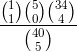(Recall that in maths, the symbols being directly next to each other just means they are being multiplied.)

Isn’t this pretty? The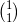is the Allure part, the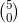is the Darks part, the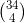is the Rest of Deck part, and we divide it by, which is the total number of possible 5 card hands out of a 40 card deck.

It’s really easy to put these into Google as well. We just type each binomial coefficient as normal, using * for multiplication and / for division:

http://prnt.sc/b772d4

The answer of 0.07047938626, when rounded up to 0.0705, matches up with the 7.05% that Rusty’s calculator gives.

Interestingly, if we take the numbers above the line, we see the top row (the 1, 5, and 34) add up to the number of cards in the Deck, and the lower row (the 1, 0, and 4) equals the size of the hand. This will always be the case, and serves as a good sanity check.

Let’s see another example. Suppose we want to find the probability of opening all 5 Exodia pieces.

• Out of 1 Left Leg, we want 1
• Out of 1 Right Leg, we want 1
• Out of 1 Left Arm, we want 1
• Out of 1 Right Arm, we want 1
• Out of 1 Exodia head, we want 1
• Out of the rest of the 35 cards, we want 0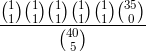As it happens,and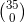are both equal to 1, so the whole thing boils down to: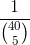Which Google will readily calculate at 0.00000151973, or 0.00015%.

Incidentally, the calculator can’t get this one properly – it rounds the answer down to 0.00%.

Drawing at least 1 Lonefire
Suppose we have 2 Lonefire in our deck, and we want to work out the chance of drawing at least 1.

At the moment, we can only calculate the chance of drawing a specific number. But not to worry. We can break this down into cases of drawing a specific numbers of Lonefires in the obvious way:

Case 1: We draw exactly 1 Lonefire, with probability: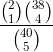This turns out to be 0.22435897435.

Case 2: We draw both Lonefires, with probability: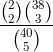This comes out to 0.01282051282.

Now we simply add the probabilities! Our total answer is 0.23717948717, or, rounding, 23.72%.

The same idea would work if we were running all 3 Lonefire. We would just split into 3 cases instead of 2.

Alternately we could use the technique in the next example.

Drawing at least 1 Ravine
Suppose we want at least 1 Dragon Ravine in our opening hand, but we have 5 in total (2 copies of Ravine and 3 Terraforming). What we could do is split into cases, drawing exactly 1,2,3,4, or 5… but that’s a lot of work. We can save some effort by thinking a little more.

There are really two possibilities here. Either we draw 0 Ravines or we draw at least 1 Ravine. These cases together have a 100% chance of happening. So, if we work out the chance of drawing 0 Ravines, we can subtract it from 100%, and that’ll leave us with the chance of drawing at least 1!

Chance of drawing 0 Ravines: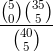Which equals 0.49335570388, i.e. 49.336%.

Drawing at least 1: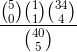100 – 49.336 = 50.66% (after rounding).

Drawing at least 1 Magician and Shark
Now let’s say we want to know the chance of drawing at least 1 Shark and either 1 or 2 Magicians (both out of 3).

Again we will break it up into cases and do each separately (at least, this is the most mindless way to do it). We just need to carefully track all of the possible combinations.

Let’s use M for magician and S for shark. We want:

1M+1S
1M+2S
1M+3S
2M+1S
2M+2S
2M+3S

I won’t do it here, but it’s entirely doable, and more importantly, it’s doable without a great deal of mental effort.

This is the way our calculator performs the calculations. It carefully runs through the different combinations that are wanted, and sums them together.

Draw cards
Actually there are some things our calculator cannot account for on its own, namely draw cards. Let’s see how to handle those.

Suppose we want to know the chance of drawing into at least 1 Dragon Ravine out of 5 like before, but this time we also have a (single) copy of Pot of Greed to help us.

There are two cases: either we open at least 1 Ravine, or we don’t open it. If we open it, we’re happy and don’t care what else we have in our hand. We worked this out earlier to be 50.66.

If we don’t open it, hope is not lost if we’ve drawn Pot of Greed. Let’s work out the chance of this scenario occurring.

Step 1: No Ravine, but 1 Pot of Greed.

This we can calculate:This works out as 0.07047938626.

Step 2: After Step 1, we then draw into Ravine from Pot of Greed.

This we can also calculate exactly the same way as we have been doing. Essentially we’re taking a hand size of 2 cards from a deck which now has 35 cards in it (since we’ve drawn 5 already).

For simplicity let’s say we want exactly 1 Ravine out of the 5 we still have in the Deck: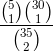This works out as 0.25210084033.

We then multiply this answer with the probability in step 1. This is because we need both steps to happen in a row. It’s like flipping a coin twice: the probability of getting 2 heads in a row is ½ multiplied by ½.

Hence our answer is: 0.0177679125, or 1.78%.

We can then add this on to the probability of opening Ravine in the first place and we get 50.66+1.78 = 52.44%.

I hope this article served to make the maths a little more transparent. Until next time!